# Community Articles

Find and share helpful community-sourced technical articles.

## Part 2: Nifi flow creation to parse new images and run the model

Labels (4)

• ### Tensorflow

pvidalCloudera Employee

# Introduction

Let's jump into tutorial 2 from my AI to Edge series!

This tutorial details the creation of a Nifi flow executing the ONNX model we trained in my last article.

More precisely we will try to feed these 3 handwritten digits and predict their value:Note: as always, all code/files referenced in this tutorial can be found on my github, here.

## Agenda

Below is an overview of the flow:As you can see, the flow is divided in the following sections:

• Section 1: Listening to a folder for new png files
• Section 2: Resizing these images to 28x28 (size used to train our model)
• Section 3: Converting these images to CSV (format used to train our model)
• Section 4: Running our predictive model

# Section 1: Listening to a folder for new png files

## Step 1: Setup a variable for the root folder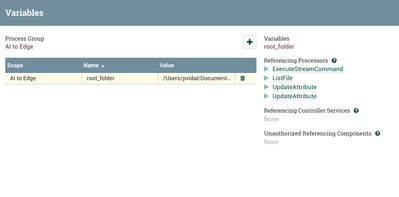This will be useful when we deploy the flow to a minifi flow. Go to your variables and create the following:

• Name: `root_folder`
• Value: `location of your download of my github`

## Step 2: List files in folder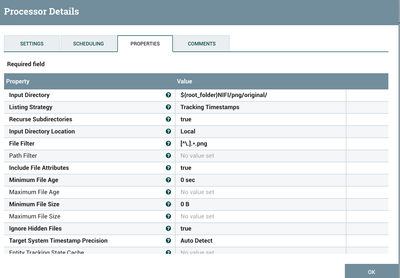Create a ListFiles processor and modify the following properties:

• Input Directory: `\${root_folder}NIFI/png/original/`
• File Filter: `[^\.].*.png`

## Step 3: Fetch files in folder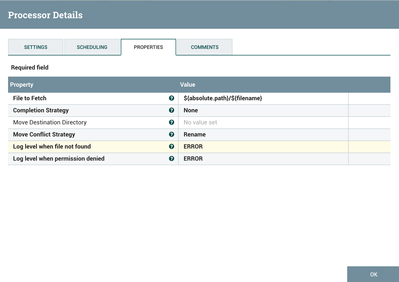Create a FetchFiles processor with default parameters.

Note: The List/Fetch paradigm is very powerful because it will allow us to continuously look for new images without reprocessing all of them. ListFiles is a stateful processor. If you're unfamiliar with the concept I encourage you to read about it on this community.

# Section 2: Resizing these images to 28x28

## Step 1: Resize Image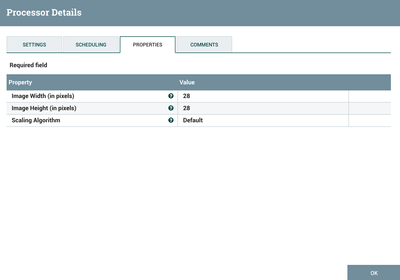Create a ResizeImage processor and modify the following properties:

• Image Width (in pixels): `28`
• Image Height (in pixels): `28`

## Step 2: Enter out attributes for resized images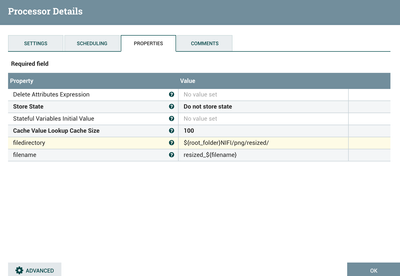Create an UpdateAttribute processor, aimed at defining the folder and filename of the resized images, by adding the following properties to the processor:

• filedirectory: `\${root_folder}NIFI/png/resized/`
• filename: `resized_\${filename}`

# Section 3: Converting these images to CSV

## Step 1: Saving modified image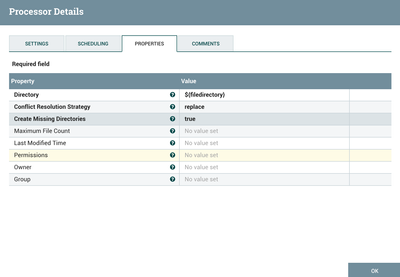Create a PutFile processor and modify the following properties to store the converted image in the resized folder:

• Directory: `\${filedirectory}`

## Step 2: Execute a python script to convert images to CSV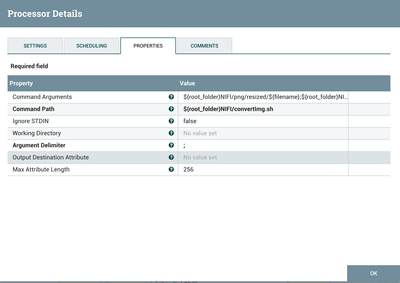In this step we will create an ExecuteStreamCommand processor that will run the `convertImg.sh` python script. The script takes the resized image file, converts it to grayscale, and converts it into an inverted CSV to match the input of our model. Below is the script itself:

```#!/usr/bin/env python3

import os,png,array
import pandas as pd
import time
import sys

from PIL import Image
from PIL import ImageOps
columnNames = list()

for i in range(784):
pixel = 'pixel'
pixel += str(i)
columnNames.append(pixel)

train_data = pd.DataFrame(columns = columnNames)
start_time = time.time()

img_name = sys.argv
img = Image.open(img_name)
img = img.convert('LA')

data = []
for y in range(28):
for x in range(28):
data.append(rawData[x,y])
print(i)
k = 0

#print data
train_data.loc[i] = [255-data[k] for k in range(784)]
csvFile = sys.argv
print(csvFile)

train_data.to_csv(csvFile,index = False)```

As you can see it expects two arguments:

• Location of the resized image (`img_name = sys.argv`)
• Location of the target CSV (`csvFile = sys.argv`)

Thus, you will modify the following properties in the ExecuteStreamCommand processor:

• Command Arguments: `\${root_folder}NIFI/png/resized/\${filename};\${root_folder}NIFI/csv/\${filename}.csv`
• Command Path: `\${root_folder}NIFI/convertImg.sh`

# Section 4: Running our predictive model

## Step 1: Enter input attributes for model execution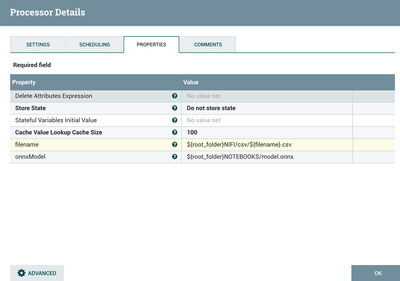Create an UpdateAttribute processor, aimed at defining the locations of the CSV file and the ONNX model, by adding the following properties to the processor:

• filename: `\${root_folder}NIFI/csv/\${filename}.csv`
• onnxModel: `\${root_folder}NOTEBOOKS/model.onnx`

## Step 2: Use python to run the model with onnxruntime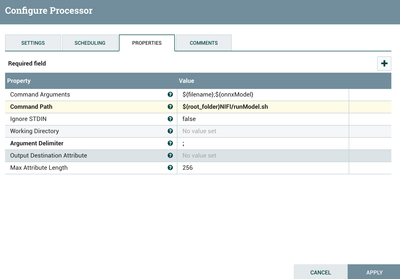In this step we will create an ExecuteStreamCommand processor that will run the `runModel.sh` python script. The script takes the CSV version of the image and run the ONNX model created in the last tutorial with this CSV as an input. Below is the script itself:

```#!/usr/bin/env python3

import onnxruntime as rt
import onnx as ox
import numpy
import pandas as pd
import shutil
import sys

X_test = test.values.astype('float32')
X_test = X_test.reshape(X_test.shape, 28, 28,1)

session = rt.InferenceSession(sys.argv)

input_name = session.get_inputs().name
label_name = session.get_outputs().name
prediction = session.run([label_name], {input_name: X_test.astype(numpy.float32)})

number = 0

for i in range(0, 9):
if (prediction[i] == 1.0):
number = i

print(number)```

As you can see it expects two arguments:

• Location of the CSV (`test=pd.read_csv(sys.argv)`)
• Location of the ONNX model (`session = rt.InferenceSession(sys.argv)`)

Thus, you will modify the following properties in the ExecuteStreamCommand processor:

• Command Arguments: `\${filename};\${onnxModel}`
• Command Path: `\${root_folder}NIFI/runModel.sh`

# Results

If you run the flow against the image in my github, you will see 3 output flowfiles, predicting the value of the handwritten digit, like shown below: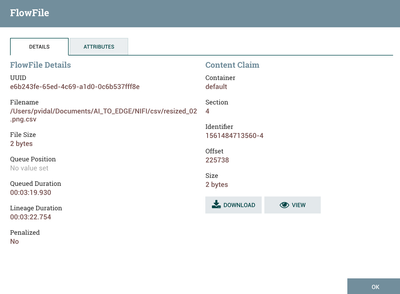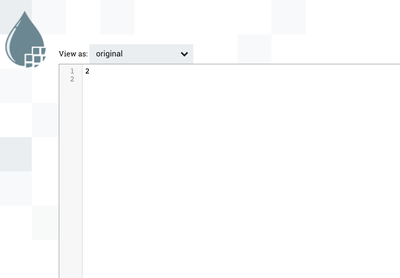2,435 Views
Take a Tour of the Community
Community Browser
Don't have an account?